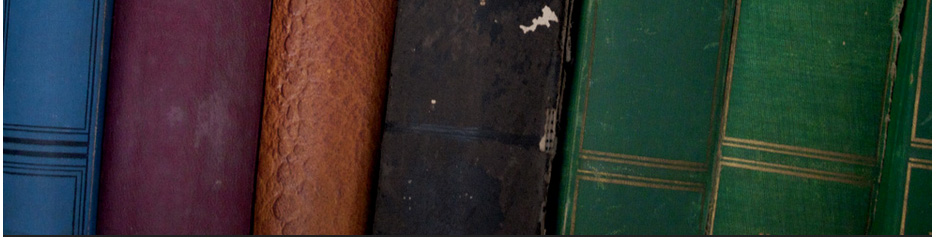## Secondary educationMaths FOR KS 4

Algebra Geometry Statistics GCSE Maths Revision
Surds Perpendicular Bisectors Collecting data - AQA National 5 Mathematics Revision Notes
Simultaneous and linear equations Vectors Representing data CFE Mathematics National 4
Solve quadratic equation Transformations Analysing data National 4 Exam Revision
Quadratic Equation Graph Loci and constructions Solving 'statistical' problem GCSE Maths
Linear Sequences and nth terms Bearings Probability GCSE Maths Revision Checklist
Laws of indices Angles, lines and polygons Histograms N5 Maths – Whole Course
Algebraic expressions and Formula Volume and surface areas, Perimeter Standard deviation Geometry (all content)
Straight line graphs Circle theorems Mean, mode and median Revision Practice for Geometry
Quadratic sequences Arcs and areas of sectors Variance and standard deviation Appendix: mathematical formulae
Index rule problems Pythagores Bivariate analysis

## GCSE Practice Questions

Algebric fractions Trigometry Sampling

## GCSE Revision

Algebra Geometry Statistics

## Formulae - GCSE Maths formulae sheet

Binomial GCSE Revision [More GCSE Revision ]

Ermias Home

HAREP The Horn of Africa Research and Knowledge Exchange Platform © 2010-2012